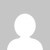# Calculator using switch case in JavaScript | Complete code

Simple take input “add”, “divide”, “multiply”, or “subtract” option form use along with 2 numbers then Calculator using switch case in JavaScript.

## Calculator using switch case in JavaScript

Simple example code user is prompted to enter an operator (either +, , *, or /) and two numbers.

``````<!DOCTYPE html>
<html>
<body>

<script>

let result;

const operator = prompt('Enter operator ( either +, -, * or / ): ');

const number1 = parseFloat(prompt('Enter first number: '));
const number2 = parseFloat(prompt('Enter second number: '));

switch(operator) {
case '+':
result = number1 + number2;
console.log(`\${number1} + \${number2} = \${result}`);
break;

case '-':
result = number1 - number2;
console.log(`\${number1} - \${number2} = \${result}`);
break;

case '*':
result = number1 * number2;
console.log(`\${number1} * \${number2} = \${result}`);
break;

case '/':
result = number1 / number2;
console.log(`\${number1} / \${number2} = \${result}`);
break;

default:
console.log('Invalid operator');
break;
}
</script>

</body>
</html
>``````

Output:

You can simply add a button to call a function like this

``````<script>
function Calculatrice(a, b, op) {

switch (op) {

case '+':
return a + b
break;
case '-':
return a - b
break;
case '*':
return a * b
break;
case '/':
return a / b
break;

}
}
console.log(Calculatrice(5, 5, '+'));

</script>``````

Output: 10

Do comment if you have any doubts or suggestions on this JS switch case topic.

Note: The All JS Examples codes are tested on the Firefox browser and the Chrome browser.

OS: Windows 10

Code: HTML 5 Version

## 1 thought on “Calculator using switch case in JavaScript | Complete code”

1.hello, how can I use the switch statement to disable the alphabet keyboard?

console.log(event);

switch (event.key) {
case “C”:
case “Backspace”:
display.textContent = “”;
break;

case “ArrowUp”:
case “ArrowDown”:
case “ArrowLeft”:
case “ArrowRight”:
break;

case “=”:
display.textContent = eval(display.textContent);
break;

case “Enter”:
display.textContent = eval(display.textContent);
break;

default:
display.textContent += event.key;
}
});

This site uses Akismet to reduce spam. Learn how your comment data is processed.